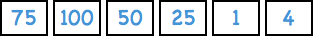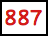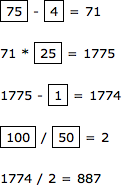## Number Countdown

Number Countdown is a game played on the popular UK television programme Countdown. The contestants are given six numbers and a three-figure target, and the aim is to try and reach the target by combining the six numbers using addition, subtraction, multiplication, and division. Only integers may be used at any stage in the calculation, and each number can only be used once.

As an example, here's a particularly hard problem. The six numbers are:and the target is:In this project we will write a Lisp program to solve any Number Countdown problem. The approach we use could be extended to solve many types of mathematical, logical, and word problems, such as problems presented in magazines and puzzle books.

Complete listing

### The approach

The typical approach to solving this type of problem is as follows:

• Construct every possible equation using the six candidate numbers combined with the four operators.
• Test each equation to see if it evaluates to the target.

However, because of the large number of possible equations, this will often need an infeasibly large amount of computing time, so an important part of the approach is to reduce the number of equations we have to consider by eliminating equations that cannot be solutions, or that duplicate an existing equation.

### Representing the problem

We will use global variables to represent the numbers, operators, and target for the problem:

```(defparameter countdown-numbers '(75 100 50 25 1 4))

(defparameter countdown-operators '(?+ ?- ?* ?/))

(defparameter countdown-target 887)```

Note that for simplicity we are going to assume that the numbers are all different. It makes the programming a bit more complicated if we want to remove this restriction.

For the operators we will define our own procedures called ?+, ?-, ?*, and ?/. More about this later.

A good starting point in solving a problem like this is to decide how we're going to represent the answer, assuming we can find it. For this problem we will use a standard Lisp expression; for example:

`(* (+ 4 1) (* 2 3))`

would be a solution for getting a target of 30 using the numbers 1, 2, 3, and 4. We can check this by doing:

```CL-USER > (eval '(* (+ 4 1) (* 2 3)))
30
```

Reducing the number of candidates

We can reduce the number of possible candidate equations by observing that:

• (+ a b) can be eliminated if b is greater than a, because it is the same as (+ b a).
• (* a b) can be eliminated if b is greater than a, because it is the same as (* b a).
• (- a b) can be eliminated if b is greater than a, because we're not interested in negative numbers.
• (* a b) can be eliminated if b is 1, as it's equivalent to a.
• (+ a b) can be eliminated if b is zero, as it's equivalent to a.
• (* a b) can be eliminated if b is zero, because zero is redundant in the expression.
• (/ a b) can be eliminated if b is zero, because division by zero will cause an error.
• (/ a b) can be eliminated unless it's an integer, as fractions are disallowed by the rules.

Many of these roughly halve the number of candidate expressions, so applying them all causes a dramatic reduction in the number of candidate expressions we have to consider.

We will implement these optimisations by defining our own version of the four arithmetic operations, called ?+, ?-, ?*, and ?/. Our versions will return nil if we want the whole expression to be eliminated:

```(defun ?+ (a b)
(if (or (null a) (null b) (< a b) (zerop a))
nil
(+ a b)))

(defun ?- (a b)
(if (or (null a) (null b) (zerop b) (< a b))
nil
(- a b)))

(defun ?* (a b)
(if (or (null a) (null b) (< a b) (= b 1) (= a 1) (= b 0) (= a 0))
nil
(* a b)))

(defun ?/ (a b)
(if (or (null a) (null b) (zerop b) (= b 1) (not (integerp (/ a b))))
nil
(/ a b)))```

Let's test them:

```CL-USER > (eval '(?* (?* (?+ 4 1) 3) 2))
30
```
```CL-USER > (eval '(?* 2 (?* (?+ 4 1) 3)))
NIL```

Next we are going to generate every possible candidate answer, consisting of every possible combination of the six numbers and four operators.

Since we are only allowed to use each number once we will need a procedure to check whether a number has already been used in an expression. We will use a procedure find-tree, which can be defined recursively as follows:

To check whether an expression contains a specified number:

• If the expression is an atom, the answer is true if the expression is the number.
• Otherwise the answer is true if we find the number in the first element of the expression, or in the rest of the expression.
In Lisp this becomes:
```(defun find-tree (number expression)
(if (atom expression) (eq number expression)
(or (find-tree number (first expression))
(find-tree number (rest expression)))))```

We can test it as follows:

```CL-USER > (find-tree 4 '(* (+ 4 1) (* 2 3)))
T

CL-USER > (find-tree 5 '(* (+ 4 1) (* 2 3)))
NIL
```

Next we define a procedure next-states to build up all possible expressions:

```(defun next-states (expression)
(let ((result nil))
(dolist (i countdown-numbers)
(if (find-tree i expression) nil
(setf result (cons (cons i expression) result))))
(if (>= (length expression) 2)
(dolist (i countdown-operators)
(let ((new (list i (first expression) (second expression))))
(if (eval new)
(setf result
(cons (cons new (rest (rest expression))) result))))))
result))```

This takes a partial expression, and generates a new list of partial expressions incorporating an additional operator or number. The expressions are added to the variable result, which is initially set to nil by the let statement.

The first half of the procedure adds each of the unused numbers to the start of expression. For example:

```CL-USER > (next-states '(1))
((4 1) (25 1) (50 1) (100 1) (75 1))```

If there are two or more items in the expression, the second half of the procedure combines the first two numbers in expression with each of the operators, and adds these to the result list. Expressions are only added if they do not eval to nil:

```CL-USER > (next-states '(4 1))
(((?- 4 1)) ((?+ 4 1)) (25 4 1) (50 4 1) (100 4 1) (75 4 1))```

The result list contains both complete expressions, such as:

`((?- 4 1))`

and partial expressions, such as:

`(25 4 1)`

The complete expressions are tested by goal-p below to see if they evaluate to the target number.

### Searching for the solution

Finally we define the routine tree-search to search for the solution:

```(defun tree-search (expressions)
(cond
((null expressions) nil)
((goal-p (first expressions)) (first expressions))
(t (tree-search
(append
(next-states (first expressions))
(rest expressions))))))```

This procedure tree-search takes a list of lists; each list is a partial or complete candidate expression. It says:

• If there are no more expressions to check, return nil.
• If the goal is satisfied for the first expression, return it.
• Otherwise, replace the first expression by the next-states of the first expression, and solve that.

Here's the procedure to check whether an expression solves the problem

```(defun goal-p (rule)
(and (= (length rule) 1)
(eq (eval (first rule)) countdown-target)))```

### Solving the original problem

Finally, let's run tree-search with our initial problem. To search all expressions we call tree-search with a list containing nil:

`CL-USER > (tree-search '(nil))`

After a few seconds the program returns the answer:

```((?/ (?- (?* (?- 75 4) 25) 1) (?/ 100 50)))
```

This corresponds to the following solution:Eureka!

### Extending the project

#### Duplicate candidates

One extension would be to remove the restriction that all the candidate numbers have to be different. The simplest way to do this is to provide them in a list, and access them by their index in the list. The indexes can be tested to make sure they are unique, but the numbers won't have to be.

Previous: Logic Mazes

Next: Eliza Chatbot